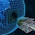### 9 balls

From Saurabh Joshi

Problem :
9 balls, 8 identical and 1 is lighter. You need to find out in 4 weights, which one is lighter. Sounds simple? Well, hold on. You are given 3 balance weight machines, out of which one is defective. You do not know which one is defective. Also, defective machine can give any outcome ( >, =, < ) irrespective of what you put on the machine. Now, can you find out which ball is lighter weighing only 4 times in total? Update:
Easier problem: A defective machine always outputs the same result. Its pointer always moves in the same direction.

Tougher problem: The defective machine gives a random result <, >, = all the time

1.Is it that the defective machine keeps giving any one of <,>,= as outputs for all inputs? or that the output randomly changes on every weighing?

The first case is simple:
divide the balls in 3 groups of 3 balls each.

Part 1:
Take the first two groups and weigh them on two machines.
Case 1: the two machine results do not match. Then use the third machine to find out which of the machines is right. Now we know the two correctly working machines. Simply use one of the correct machines for the second part.
Case 2: The two readings match and the readings are grp 1 > grp 2 or grp 2 > grp 1. Take one of these machines and interchange grp 1 and grp 2. If the machine keeps giving the same output, it is defective. Else it is not.
Case 3: The two readings match and are grp 1 = grp 2. Then take grp 3 and weigh it against grp 1. If the machine still says grp 1 = grp 3 then it is defective. Else it is not.

Part 2: In part 1, in each case we know exactly which machine is defective and also which group is the lightest. (The group measured to be light in case one of groups is light, else grp 3).
Now take two two from the lighter group and use a correct machine to weigh. If the two balls have same weight, then the third ball is the lighter one, else the lighter ball is found out on the machine.

On the other hand, if the defective machine can keep giving any random output, the problem changes (and I think becomes more complex).

2.Nice solution Prathmesh...

I think your assumption is justified... I should have made it clear in the post..

Thanx...

BTW, Even in the more complex case, it can be done in 4 weighings. I don't expect anyone to get it though. :P
http://sbjoshi.wordpress.com/2009/10/09/puzzle-9-balls/

3.New solution that I thought of when I was discussing this problem.

Divide the set into three sets (each of three balls) say S1, S2, S3.

Weigh S1 vs S2 on first scale. Then weigh S3 v S1 on first scale. Note that S1 has its side changed now. Now,
Case1: If S1 has the lighter ball and first scale is good, then the pointer should switch directions.
Case2: If S2 has the lighter ball and first scale is good, then the pointer should move in first weighing and show equal in second weighing.
Case3: If S3 has the lighter ball and the first scale is good, then the pointer should move in second weighing and show equal in first weighing.

So, in two weighings, we find if the first scale is defective or not. Now in 2 more, we can find the lighter ball using a good scale. :)

1.The defective scale can trick you probabilistically. Eventhough it gave consistent solutions and behaved well in first 2 weighings, it can misbehavr in the subsequent weighings.

4.This is a strictly better solution.
You no longer need the third machine.
Kudos!

5.